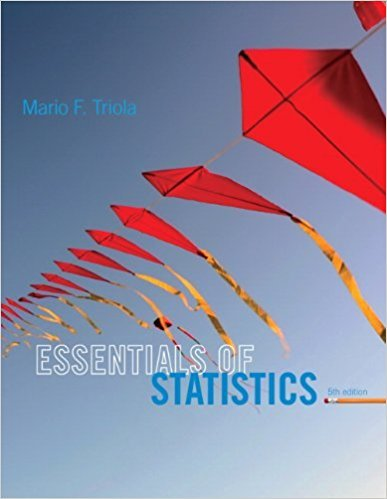×
×

# Solutions for Chapter 3.3: Measures of Variation## Full solutions for Essentials of Statistics | 5th Edition

ISBN: 9780321924599Solutions for Chapter 3.3: Measures of Variation

Solutions for Chapter 3.3
4 5 0 377 Reviews
25
1
##### ISBN: 9780321924599

This expansive textbook survival guide covers the following chapters and their solutions. This textbook survival guide was created for the textbook: Essentials of Statistics, edition: 5. Chapter 3.3: Measures of Variation includes 46 full step-by-step solutions. Since 46 problems in chapter 3.3: Measures of Variation have been answered, more than 15009 students have viewed full step-by-step solutions from this chapter. Essentials of Statistics was written by and is associated to the ISBN: 9780321924599.

Key Statistics Terms and definitions covered in this textbook
• a-error (or a-risk)

In hypothesis testing, an error incurred by failing to reject a null hypothesis when it is actually false (also called a type II error).

• Alias

In a fractional factorial experiment when certain factor effects cannot be estimated uniquely, they are said to be aliased.

• Binomial random variable

A discrete random variable that equals the number of successes in a ixed number of Bernoulli trials.

• Categorical data

Data consisting of counts or observations that can be classiied into categories. The categories may be descriptive.

• Cause-and-effect diagram

A chart used to organize the various potential causes of a problem. Also called a ishbone diagram.

• Central tendency

The tendency of data to cluster around some value. Central tendency is usually expressed by a measure of location such as the mean, median, or mode.

• Chi-square (or chi-squared) random variable

A continuous random variable that results from the sum of squares of independent standard normal random variables. It is a special case of a gamma random variable.

• Components of variance

The individual components of the total variance that are attributable to speciic sources. This usually refers to the individual variance components arising from a random or mixed model analysis of variance.

• Conditional mean

The mean of the conditional probability distribution of a random variable.

• Conditional probability mass function

The probability mass function of the conditional probability distribution of a discrete random variable.

• Conidence coeficient

The probability 1?a associated with a conidence interval expressing the probability that the stated interval will contain the true parameter value.

• Contrast

A linear function of treatment means with coeficients that total zero. A contrast is a summary of treatment means that is of interest in an experiment.

• Correlation matrix

A square matrix that contains the correlations among a set of random variables, say, XX X 1 2 k , ,…, . The main diagonal elements of the matrix are unity and the off-diagonal elements rij are the correlations between Xi and Xj .

• Defect concentration diagram

A quality tool that graphically shows the location of defects on a part or in a process.

• Deming

W. Edwards Deming (1900–1993) was a leader in the use of statistical quality control.

• Experiment

A series of tests in which changes are made to the system under study

• Exponential random variable

A series of tests in which changes are made to the system under study

• Gamma random variable

A random variable that generalizes an Erlang random variable to noninteger values of the parameter r

• Generator

Effects in a fractional factorial experiment that are used to construct the experimental tests used in the experiment. The generators also deine the aliases.

• Geometric random variable

A discrete random variable that is the number of Bernoulli trials until a success occurs.

×